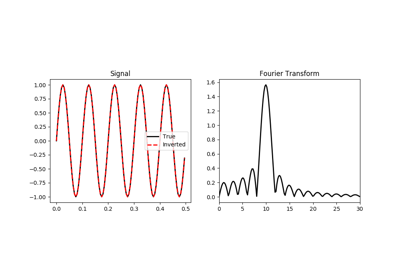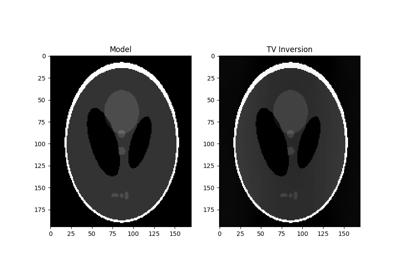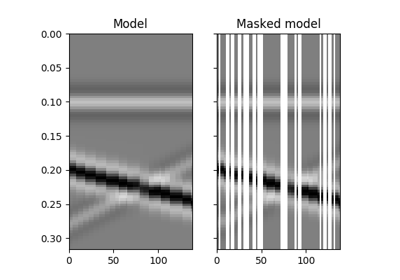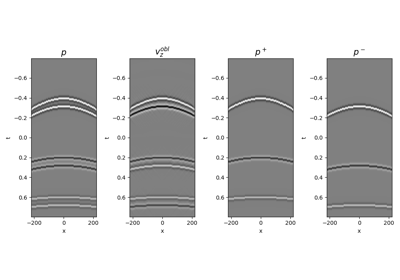# pylops.signalprocessing.FFT2D¶

class pylops.signalprocessing.FFT2D(dims, dirs=(0, 1), nffts=(None, None), sampling=(1.0, 1.0), dtype='complex128')[source]

Two dimensional Fast-Fourier Transform.

Apply two dimensional Fast-Fourier Transform (FFT) to any pair of axes of a multi-dimensional array depending on the choice of dirs. Note that the FFT2D operator is a simple overload to the numpy numpy.fft.fft2 in forward mode and to the numpy numpy.fft.ifft2 in adjoint mode, however scaling is taken into account differently to guarantee that the operator is passing the dot-test.

Parameters: dims : tuple Number of samples for each dimension dirs : tuple, optional Pair of directions along which FFT2D is applied nffts : tuple, optional Number of samples in Fourier Transform for each direction (same as input if nffts=(None, None)) sampling : tuple, optional Sampling steps dy and dx dtype : str, optional Type of elements in input array ValueError If dims has less than two elements, and if dirs, nffts, or sampling has more or less than two elements.

Notes

The FFT2D operator applies the two-dimensional forward Fourier transform to a signal $$d(y,x)$$ in forward mode:

$D(k_y, k_x) = \mathscr{F} (d) = \int \int d(y,x) e^{-j2\pi k_yy} e^{-j2\pi k_xx} dy dx$

Similarly, the two-dimensional inverse Fourier transform is applied to the Fourier spectrum $$D(k_y, k_x)$$ in adjoint mode:

$d(y,x) = \mathscr{F}^{-1} (D) = \int \int D(k_y, k_x) e^{j2\pi k_yy} e^{j2\pi k_xx} dk_y dk_x$

Both operators are effectively discretized and solved by a fast iterative algorithm known as Fast Fourier Transform.

Attributes: shape : tuple Operator shape explicit : bool Operator contains a matrix that can be solved explicitly (True) or not (False)

Methods

 __init__(self, dims[, dirs, nffts, …]) Initialize this LinearOperator. adjoint(self) Hermitian adjoint. cond(self, \*\*kwargs_eig) Condition number of linear operator. conj(self) Complex conjugate operator div(self, y[, niter]) Solve the linear problem $$\mathbf{y}=\mathbf{A}\mathbf{x}$$. dot(self, x) Matrix-matrix or matrix-vector multiplication. eigs(self[, neigs, symmetric, niter]) Most significant eigenvalues of linear operator. matmat(self, X) Matrix-matrix multiplication. matvec(self, x) Matrix-vector multiplication. rmatvec(self, x) Adjoint matrix-vector multiplication. transpose(self) Transpose this linear operator.

## Examples using pylops.signalprocessing.FFT2D¶Fourier TransformTotal Variation (TV) Regularization11. Seismic regularization12. Seismic wavefield decomposition